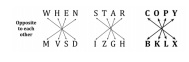# RRB ALP 2018 Practice Test Papers | Reasoning Questions (Day-24)

Dear Aspirants, Here we have given the Important RRB ALP & Technicians Exam 2018 Practice Test Papers. Candidates those who are preparing for RRB ALP 2018 can practice these Reasoning Questions to get more confidence to Crack RRB 2018 Examination.

[WpProQuiz 1880]

Click “Start Quiz” to attend these Questions and view Solutions

1. 22, 30, 42, 58,?

(A) 50

(B) 62

(C) 30

(D) 78

1. 2, 3, 5, 8, 13,?

(A) 21

(B) 19

(C) 26

(D) 33

1. Introducing Raman, Sohan said, “He is the only son of my father’s only son.” How is Sohan related to Raman?

(A) Niece

(B) Father

(C) Aunt

1. JKP, KLQ, LMR, MNS,?

(A) NOT

(B) NPT

(C) PNS

(D) MPS

1. In a certain code, ‘WHEN’ is written as ‘MVSD’ and ‘STAR’ is written as ‘IZGH’. How is ‘COPY’ written in the same code?

(A) BLKX

(B) BKXL

(C) KBLX

(D) None of these

1. Which of the following is different from the others?

(A) AF

(B) JN

(C) LQ

(D) WB

1. Select the odd one out.

(A) Playing

(B) Eating

(C) Walking

(D) Sleeping

1. Choose the pair, which is different from the rest.

(A) 36 – 63

(B) 87 – 78

(C) 35 – 51

(D) 44 – 44

1. If * stands for ‘addition’,/ stands for ‘subtraction’, + stands for ‘multiplication’ and – stands for ‘division’, then 20 * 8/ 8 – 4 + 2 =?

(A) 24

(B) 25

(C) 64

(D) 8

1. Man: Lip:: Bird:?

(A) Bush

(B) Grass

(C) Forest

(D) Beak

The pattern of the number series is as follows: 22 + (4 × 2) = 22 + 8 = 30

30 + (4 × 3) = 30 + 12 = 42

42 + (4 × 4) = 42 + 16 = 58

58 + (4 × 5) = 58 + 20 = 78

The sum of two consecutive terms of the series gives the next term as: 2 + 3 = 5

3 + 5 = 8

5 + 8 = 13

8 + 13 = 21

Sohan’s father’s only son means Sohan himself. Thus, Raman is the son of Sohan and Sohan is the father of Raman.

In the given series, letters in each term consist of their predecessor. Hence, the required term is (M + 1) (N + 1) (S + 1), i.e. NOT.The following figures describe the logic used in the given encoding:

By following the English alphabet letter position, the second letter is +5 from the first letter in all the options, except ‘JN’.

All others show the physical activities of a person, except sleeping.

All others are written in reverse order, except 35 – 51.

If we put the correct symbols, the equation “20 * 8/ 8 – 4 + 2” would be written as “20 + 8 – 8 /4 * 2”. This would be calculated as: = 20 + 8 – 2 * 2 = 20 + 8 – 4 = 24

Lip is a human body part while the beak is a bird body part.

RRB ALP 2018 Practice Test Papers | Reasoning Questions (Day-1)

RRB ALP 2018 Practice Test Papers | Reasoning Questions (Day-2)

RRB ALP 2018 Practice Test Papers | Reasoning Questions (Day-3)

RRB ALP 2018 Practice Test Papers | Reasoning Questions (Day-4)

RRB ALP 2018 Practice Test Papers | Reasoning Questions (Day-5)

RRB ALP 2018 Practice Test Papers | Reasoning Questions (Day-6)

RRB ALP 2018 Practice Test Papers | Reasoning Questions (Day-7)

RRB ALP 2018 Practice Test Papers | Reasoning Questions (Day-8)

RRB ALP 2018 Practice Test Papers | Reasoning Questions (Day-9)

RRB ALP 2018 Practice Test Papers | Reasoning Questions (Day-10)

RRB ALP 2018 Practice Test Papers | Reasoning Questions (Day-11)

RRB ALP 2018 Practice Test Papers | Reasoning Questions (Day-12)

RRB ALP 2018 Practice Test Papers | Reasoning Questions (Day-13)

RRB ALP 2018 Practice Test Papers | Reasoning Questions (Day-14)

RRB ALP 2018 Practice Test Papers | Reasoning Questions (Day-15)

RRB ALP 2018 Practice Test Papers | Reasoning Questions (Day-16)

RRB ALP 2018 Practice Test Papers | Reasoning Questions (Day-17)

RRB ALP 2018 Practice Test Papers | Reasoning Questions (Day-18)

RRB ALP 2018 Practice Test Papers | Reasoning Questions (Day-19)

RRB ALP 2018 Practice Test Papers | Reasoning Questions (Day-20)

RRB ALP 2018 Practice Test Papers | Reasoning Questions (Day-21)

RRB ALP 2018 Practice Test Papers | Reasoning Questions (Day-22)

RRB ALP 2018 Practice Test Papers | Reasoning Questions (Day-23)